# (GMAT 800) Data Sufficiency Quadratic Equations with Explanation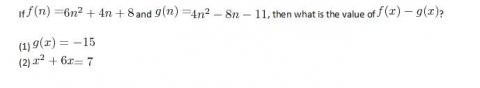A.    Statement (1) ALONE is sufficient, but statement (2) alone is not sufficient.
B.    Statement (2) ALONE is sufficient, but statement (1) alone is not sufficient.
C.    BOTH statements TOGETHER are sufficient, but NEITHER statement ALONE is sufficient.
D.    EACH statement ALONE is sufficient.
E.    Statements (1) and (2) TOGETHER are NOT sufficient to answer the question asked, and additional data are needed.

Explanation:
This question asks whether the information in one or both statements is sufficient to find a specific value. But we don't actually have to determine the value, just know that it can be determined.

(1) Quadratic equations have two solutions. However, sometimes those two solutions are identical with each other. If they are different, then solving this equation will lead to two possible values of x, and presumably also two possible values of f(x), so this would not be sufficient. But if they are the same, i.e. if there's really only one solution to this equation, then we would definitely know x, definitely know f(x), and definitely know f(x) - g(x). We have to plug in to find out how many solutions there are.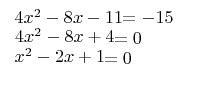Either by factoring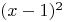or by using the quadratic equation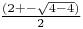, we see that this equation has a double root, 1, the two solutions are in fact identical.  Since we know exactly what x is, we also can calculate f(x), and this is sufficient to calculate the value of f(x)-g(x). (33, but don't bother doing the calculation on the exam).
SUFFICIENT

(2) Here we are given a quadratic equation, which we could solve. Factoring, we'll find two roots: -7 and 1. So it would seem that this is not sufficient to know the value of f(x)-g(x).

However, don't despair too quickly. Although this isn't enough information to positively state the value of f(x) or g(x), it is enough to determine the value of f(x)-g(x). Subtracting the two equations, we get: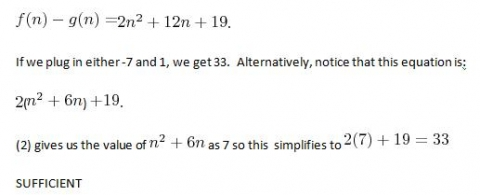The credited response is D - each statement alone is sufficient.Grockit, an online test  prep game, is the smartest way to study for your test. It's adaptive, fun and finds the right teacher for you. Grockit’s analytic capabilities and adaptive technology identifies students' strengths and weaknesses, focusing the student's study time.  Students can practice in adaptive solo games, play social learning games with peers, and work with experts that match their specific needs.1. Complete GMAT RC Questions in less than 1 minute and 50 seconds
3. Take Notes Effectively
4. Collect and Interpret Facts
5. Speed up Summary Creation
6. Remember Information
7. Question the Author

12. Learn to Answer GMAT organization of passage Question
13. Learn to identify the style/tone or attitude of the author

Mastering GMAT Critical ReasoningAfter you read F1GMAT’s Mastering GMAT Critical Reasoning Guide, you will learn:

How to overcome flawed thinking in GMAT Critical Reasoning?

How to spot Inconsistencies in Arguments

How to eliminate out of scope answer choices using Necessary and Sufficient Conditions

How to Paraphrase GMAT Critical Reasoning Question

How to Answer Assumption Question Type

How to Answer Conclusion Question Type

How to Answer Inference Question Type

How to Answer Strengthen Question Type

How to Answer Weaken Question Type

How to Answer bold-faced and Summary Question Types

How to Answer Parallel Reasoning Questions

How to Answer the Fill in the Blanks Question

Get F1GMAT's Newsletters (Best in the Industry)

• Ranking Analysis
• Post-MBA Salary Trends
• Post-MBA Job Function & Industry Analysis
• Post-MBA City Review
• MBA Application Essay Tips
• School Specific Essay Tips
• GMAT Preparation Tips